# Comparing Logarithms With Different Bases

Logarithms are not hard to work with when only one base is involved (as in most real-life problems); but they can be challenging when each log has a different base. Here, we’ll look at a few problems in which we have to compare logarithms with different bases, showing various strategies.

The questions came from Debarghya in mid-June. The first two came together, but I’ll separate them so we can follow them more easily.

## Problem 1: Arranging logarithms in order

Here is the first:

I tried to solve these problems:

1.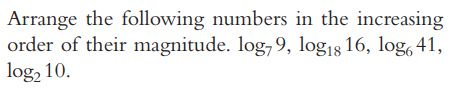I don’t have any clue how to compare logarithmic numbers.

This would be easy enough to answer with a calculator; the four numbers are, respectively, about 1.1292, 0.9592, 2.0726, and 3.3219, and you can just put those numbers in order. But what if you can’t use a calculator?

Hi, Debarghya.

You have some good questions!

There are several things you could do to compare logs; my normal first thought would be to use the change of base formula,

logb(x) = loga(x)/loga(b)

and try to write them with a common base and common denominator; but that doesn’t help much, if at all, here.

My next thought works: Estimate each log by finding two integers it must be between. For example, since 7 < 9 < 49, log7(7) < log7(9) < log7(49), so 1 < log7(9) < 2. In this case, that will be all the precision you need.

I used the change of base formula to calculate the values above; but to do that without a calculator, we would want to somehow relate them all to values we could calculate, and I couldn’t see a way to do that. I’ve seen problems where it would have been helpful.

So it looked like some sort of estimation might help, and when I did that in the roughest way I could, I saw that the numbers were carefully chosen to make this possible. As I showed, we can estimate the first number by finding two successive powers of 7 that 9 lies between, namely $$7^1=7$$ and $$7^2=49$$, which led to a pair of successive integers that the log is between, namely, 1 and 2.

Debarghya replied with a small error followed by good work:

I don’t understand how could I estimate those two integers? Since base is 7 so, I have to take some powers of 7 between which 9 lies. Here I take two smallest powers of 7 between which 9 lies.  But for log1816 I need to take 18 and 182between which 16 lies. but that results in same interval 1 and 2.

I simplified log1816 and I got 4/(1+2log23). Considering 3 is between 2 and 4  I found two limiting values of 4/(1+2log23) as 1.33 and 0.8. How I compare log1816 and log79?

Then I thought about a different way of getting those numbers. plz see the attachment. Did you mean this way?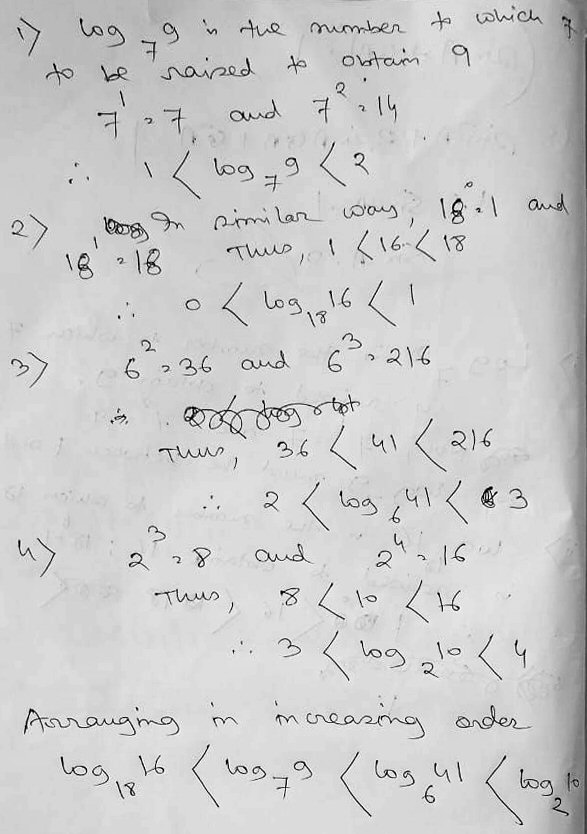The first attempt went awry when he chose two wrong powers of 18, making it seem not to work; it is not true that $$18^1<16<18^2$$, but rather $$18^0=1<16<18=18^1$$. It is not uncommon for a tiny error to lead us away from a good method!

The second paragraph is a correct simplification using base 2: $$\log_{18}(16)=\frac{\log_2(16)}{\log_2(18)}=\frac{\log_2(2^4)}{\log_2(2^1\cdot3^2)}=\frac{4}{1+2\log_2(3)}$$ Debarghya evidently used the fact that $$1<\log_2(3)<2$$ to find that $$0.8=\frac{4}{5}<\frac{4}{1+2\log_2(3)}<\frac{4}{3}\approx1.33.$$ That estimate could conceivably help, but we don’t know that $$\log_7(9)>1.33$$. (In fact, it is not; we would need a tighter estimate of $$\log_{18}(16)$$.)

But the handwritten work is good, and is just what I had suggested.

I responded:

It is not true that 16 is between 18 and 182. You corrected that in the attachment! Your attempt to simplify is more or less the method I said I abandoned because it didn’t lead to anything useful.

The “different” way is exactly what I suggested. Good work.

I like seeing both successful and unsuccessful attempts, and in fact I like showing them here, because “failures” are part of the process of problem-solving. It’s particularly important in this example to understand that no one method would work for any set of numbers they could give; these were chosen to be particularly easy – if you happen to think the right way.

Lesson 1: Make a hopeful guess that a very easy method might work! (If it doesn’t, you can either refine it, or rethink it entirely. But don’t give up too soon!)

## Problem 2: Comparing a logarithmic expression to zero

Here is the second problem, part of the same original question:

2.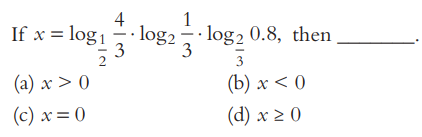To solve above problem I got these informations in a book: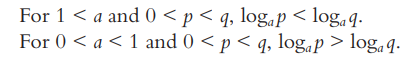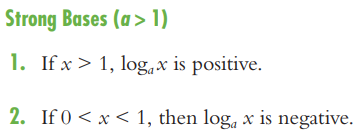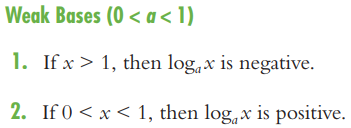I checked these conditions using log calculator and I found them true. But could I prove them algebraically? I searched over internet but I got no formal proof. only these statements and examples are given. I think that these conditions are important to compare log numbers. But they are not discussed in detail anywhere. I need your help doctor.

It can help to memorize many special-purpose rules like these, and many teachers around the world teach that. I don’t like trusting my memory too much; being able to prove them can either make them more memorable, or make them unnecessary. We’ll see a couple ways to do that.

All you need is to find the sign of each factor, which is what the facts you found do, and then combine them. I didn’t use those facts directly, because I don’t have them memorized, but in effect I derived them myself, by writing each of the three logs in terms of a known positive log.

For example,

log1/2(4/3) = log(4/3) / log(1/2) = log(4/3) / -log(2)

which is negative because both 4/3 and 2 are greater than 1, so their base-ten logs are positive.

Do the same thing with the other two logs.

Here I first used the change-of-base formula, then rewrote the denominator so that each log is positive and all signs are explicitly visible. Now I know that the sign of this expression is negative.

I could equivalently use the less-familiar fact that

log1/b(x) = -logb(x)

which is “obvious” from the change of base formula, or from the definition of a log.

This is another formula I don’t really have memorized; I just know it exists, and rederive it as needed, which is what I mean by scare-quote “obvious”. Here is the first derivation I mentioned: $$\log_{\frac{1}{b}}(x)=\frac{\log(x)}{\log{\frac{1}{b}}}=\frac{\log(x)}{-\log{b}}=-\log_b(x)$$

Here is the derivation from the definition of the logarithm: $$y=\log_{\frac{1}{b}}(x)\;\Rightarrow\;\left(\frac{1}{b}\right)^y=x\;\Rightarrow\;b^{-y}=x\;\Rightarrow\;y=-\log_b(x)$$

You can prove the theorems you quote using the same methods. And they can be memorized by visualizing the graphs of logs with base greater than or less than 1: a log with base greater than 1 is an increasing function, and a log with (positive) base less than 1 is a decreasing function.

I hope that helps.

Here is a proof of the “second rule for strong bases” using the change-of-base formula:

$$\text{Suppose that }a>1\text{. Then}\\0<x<1\;\Rightarrow\;\log_a(x)=\frac{\log(x)}{\log(a)}=\frac{-\log\left(\frac{1}{x}\right)}{\log(a)}<0\\\text{because }\frac{1}{x}>1\text{ and }a>1.$$

Here is a proof of the “first rule for weak bases” using the definition of a logarithm:

$$\text{Suppose that }0<a<1\text{ and }x>1.\text{ Then}\\\log_a(x)=y\;\Rightarrow\;1<x=a^y=\left(\frac{1}{a}\right)^{-y}\\\;\Rightarrow\;-y>0\;\Rightarrow\;y<0\;\Rightarrow\;\log_a(x)<0\\\text{because }x>1\text{ and }\frac{1}{a}>1.$$

And here are the graphs demonstrating all the rules: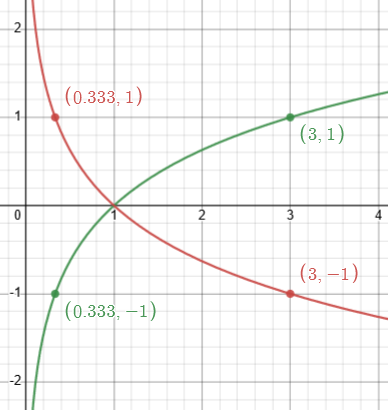In green is $$y=\log_3(x)$$, which is an increasing function because the base is greater than 1; in red is $$\log_{1/3}(x)$$, which is a decreasing function because the base is positive but less than 1. We can see, using informal terms, that

• the log of a big number to a big base is positive,
• the log of a small number to a big base is negative,
• the log of a big number to a small base is negative, and
• the log of a small number to a small base is positive.

Debarghya replied,

This case is absolutely clear and very interesting idea is to visualize graph. For base > 1 we have rising curve and for base < 1 we have decaying curve.

log1/2(4/3) gives decaying curve so -ve

log2(1/3) gives rising curve so -ve

log2/3(0.8) gives decaying curve so +ve

thus, x>0.

Here are the three points on the three curves $${\color{DarkGreen}{f\left(x\right)=\log_{\frac{1}{2}}\left(x\right)}}$$, $${\color{Red}{g\left(x\right)=\log_{2}\left(x\right)}}$$, and $${\color{Blue}{h\left(x\right)=\log_{\frac{2}{3}}\left(x\right)}}$$, which we can visualize without needing an exact graph: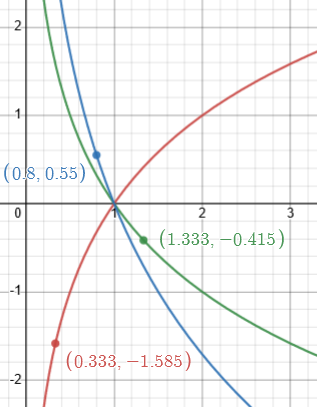We have $$x>1$$ on a falling curve, therefore negative; $$x<1$$ on a rising curve, therefore negative; and $$x<1$$ on a falling curve, therefore positive.

I responded:

The specific work I did, not using the graphs, was like this:

log1/2(4/3) = log(4/3) / log(1/2) = log(4/3) / -log(2) < 0

log2(1/3) = log(1/3) / log(2) = -log(3) / log(2) < 0

log2/3(0.8) = log(4/5) / log(2/3) = -log(5/4) / -log(3/2) > 0

So the product is positive.

But your way is quicker, as long as you trust it! Good work.

(Curiously, this means that both (a) and (d) are correct answers!)

I say this because if $$x>0$$, then it is also true that $$x\ge0$$. The former, though, is the more specific fact.

Lesson 2: A visual sense of how logs work, from their graphs, can be very powerful. (But be sure to cover all cases, not just the usual greater-than-one bases that we get used to.)

## Problem 3: Comparing two fractional logarithms

Now Debarghya submitted a third problem, which looks complicated, but turns out to be easy if you look at it in the right way (and know a few tricks):

I have found a slightly different type of question: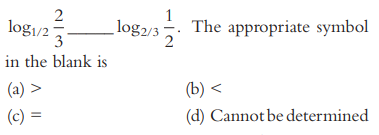I cannot solve it because there is fractional case. We cannot use how much power of 1/2 is 2/3 or how much power of 2/3 is 1/2. How to approach this?

Moreover both values are positive. So, we cannot compare them from their signs.

The last comment is a good one: Debarghya has learned how to quickly determine the sign of such an expression, and hoped this would be an easy problem similar to our first, if 0 lies between the two numbers. Alas, it does not. So we need a different approach.

I would start by rewriting both logs using the change of base formula, which I mentioned before, perhaps using 2 as the new base.

One thing you’ll notice is that the two numbers are reciprocals of one another. When is a number greater than its reciprocal?

Or you might use a common denominator to compare them.

If you show your actual work, I’ll be able to say more.

I’ve given a couple hints whose relevance won’t be seen until the work has been done. (Why use 2 as the base? Just because it’s small, and there are some 2’s in the problem.)

Debarghya did the work I suggested: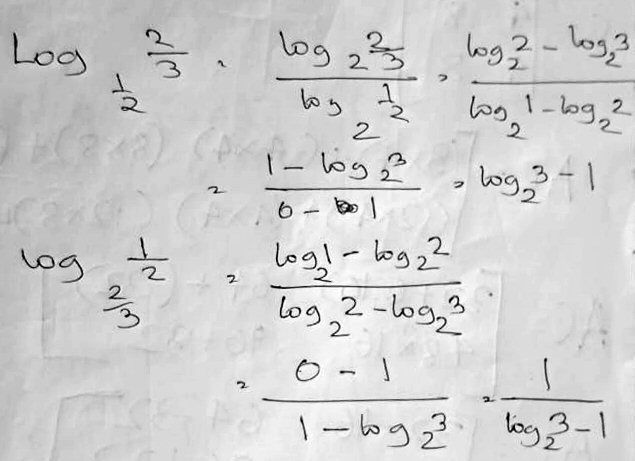How could I tell which is bigger?

As I’d said, these two numbers are reciprocals (and using base 2 did help). Is there a nice way to compare them? I’d hinted at two possibilities, and now I’ll suggest a third.

I responded:

Good work.

Now use the kind of thinking we did for the ordering problem: What integers does log2(3) – 1 lie between?

Then, what does that tell you about how it compares to its reciprocal?

I asked you before, “When is a number greater than its reciprocal?” That is, under what conditions is x > 1/x? Did you think about that? (Given our context, you can assume x > 0.)

Debarghya said,

Here is what I did:

log2(3) lies between 2 and 4

so, 1 < log2(3) – 1 < 3.

so, 1 > 1/(log2(3) – 1) > 1/3.

thus, log2(3) – 1 is greater than 1/(log2(3) – 1). is it right?

A number is greater than its reciprocal when it lies between 1 to ∞ or 0 to -1.

Since log2(3) – 1 lies between 1 and 3 i.e., between 1 to ∞ so it must be greater than its reciprocal. from this perspective also we can see that log2(3) – 1 is greater than 1/(log2(3) – 1).

Thus log2/3(1/2) is greater than log1/2(2/3). is it correct?

I’d intended to say, “what consecutive integers does $$\log_2(3)$$ lie between”, as in our first problem. I suspect that Debarghya meant to say that 3 lies between 2 and 4, which are powers of 2, and forgot to take their logs! This resulted in a wrong interval, so that the subsequent conclusions are wrong; but he is right about when a number is greater than its reciprocal. Since we know both numbers of interest are positive, we can solve $$x > \frac{1}{x}$$ under that condition by multiplying both sides by (the positive number) x, to get $$x^2>1$$, so that $$x>1$$. If x were negative, we would get $$x^2<1$$, so that $$-1<x<0$$. We can also see this on a graph of $$f(x)=x$$ (green) and $$g(x)=\frac{1}{x}$$ (red):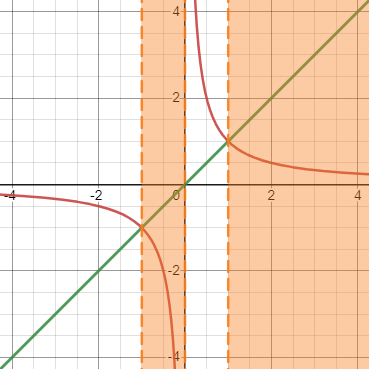I replied:

The basic ideas are there, but you skipped a step that resulted in a major error:

log2(3) lies between 2 and 4

so, 1 <  log2(3) – 1 < 3.

so, 1 > 1/(log2(3) – 1) > 1/3.

Since 3 lies between 2 and 4, that is, 21 and 22, therefore log2(3) lies between 1 and 2.

This changes everything.

The reality is that log2(3) – 1 ≈ 0.58496, and 1/(log2(3) – 1) ≈ 1.7095, contrary to your claims.

Somehow, though, you seem to have forgotten which log equals which expression, so that you accidentally got the correct final answer. In claiming that log2(3) – 1 is greater than 1/(log2(3) – 1), you are saying that log1/2(2/3) is greater than log2/3(1/2), not what you say at the end.

Debarghy finished the work:

I had mistakenly written log2(3) lies between 2 and 4. Instead I wanted to write the following

1 < log2(3) < 2

0 < log2(3) – 1 < 1

So, 1/0 > 1/(log2(3) – 1) > 1. thus 1/(log2(3) – 1 > 1 and log2(3) – 1 < 1.

Thus, log2(3) – 1 is less than 1/(log2(3) – 1).

Thus log1/2(2/3) is less than log2/3(1/2). Is it right?

Moreover, a number is greater than its reciprocal when it lies between 1 to ∞ or 0 to -1.

Since log2(3) – 1 lies between 0 and 1 so it must be lesser than its reciprocal. from this perspective also we can see that log2(3) – 1 is less than 1/(log2(3) – 1). Is it ok now?

Once we correctly see that $$\log_{1/2}\left(\frac{2}{3}\right)=\log_2(3)-1<1$$, we know that its reciprocal is greater than 1, so that the former is smaller.

I concluded:

Yes, these are the corrections you needed.

Lesson 3: When you near the end of a problem, don’t toss away your brain and start running to the finish line! Check everything you do.

We could have done the work more quickly without simplifying (following my hints), by just seeing that $$\log_{\frac{1}{2}}\left(\frac{2}{3}\right)$$ is between 0 and 1 (because this log is a decreasing function, and $$\frac{1}{2}<\frac{2}{3}<1$$, so $$1>\log_{\frac{1}{2}}\left(\frac{2}{3}\right)>0$$). As a result, it is less than its reciprocal.

## Generalizing the problem

Since the problem has such a simple form, I want to generalize it: Under what conditions is $$\log_b(a)>\log_a(b)$$?

One approach would be to examine the difference: $$\log_b(a)-\log_a(b)=\frac{\log(a)}{\log(b)}-\frac{\log(b)}{\log(a)}=\frac{(\log(a))^2-(\log(b))^2}{\log(a)\log(b)}$$

The numerator is positive when $$|\log(a)|>|\log(b)|$$, and the denominator when $$\log(a)$$ and $$\log(b)$$ have the same sign. So $$\log_b(a)>\log_a(b)$$ when either

• $$|\log(a)|>|\log(b)|$$ and $$\log(a)$$ and $$\log(b)$$ have the same sign, or
• $$|\log(a)|<|\log(b)|$$ and $$\log(a)$$ and $$\log(b)$$ have opposite signs.

Otherwise, $$\log_b(a)<\log_a(b)$$.

In our case, with $$a=\frac{2}{3}$$ and $$b=\frac{1}{2}$$, both are less than 1, so their logs are both negative (same sign), and $$|\log(a)|<|\log(b)|$$ (imagine the graph of $$\log(x)$$ as below):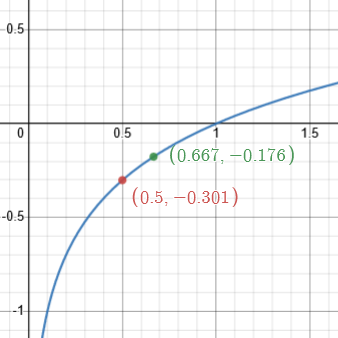So $${\color{Green}{\log_{\frac{1}{2}}\left(\frac{2}{3}\right)}}<{\color{Red}{\log_{\frac{2}{3}}\left(\frac{1}{2}\right)}}$$

As we saw before, the numbers are, respectively, about 0.5850 and 1.7095, so this is correct. And here they are on their graphs: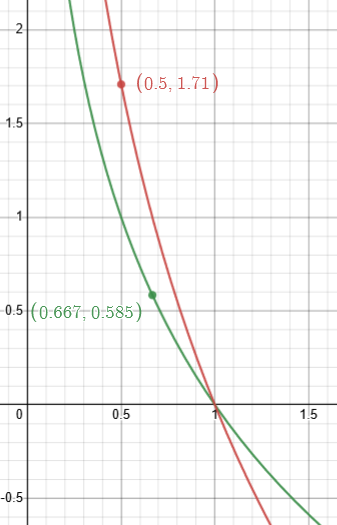This site uses Akismet to reduce spam. Learn how your comment data is processed.